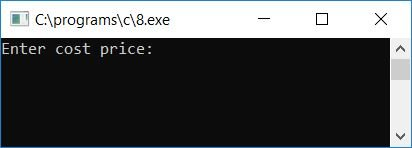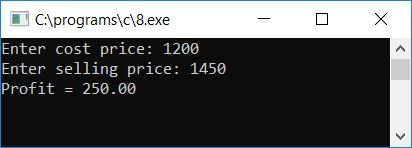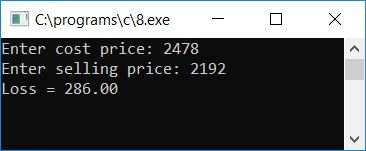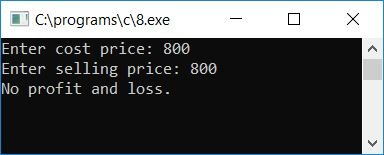# C Program to Find Profit and Loss

In this article, we will learn about how to create a program in C that will ask the user to enter the cost price and selling price of any item to calculate and print the profit or loss on that item.

```#include<stdio.h>
#include<conio.h>
int main()
{
float sp, cp, pf, ls;
printf("Enter cost price: ");
scanf("%f", &cp);
printf("Enter selling price: ");
scanf("%f", &sp);
if(sp>cp)
{
pf = sp-cp;
printf("Profit = %.2f", pf);
}
else if(cp>sp)
{
ls = cp-sp;
printf("Loss = %.2f", ls);
}
else
printf("No profit and loss.");
getch();
return 0;
}```

As the above program was written in the Code::Blocks IDE, here is the snapshot of the first sample run:Now supply the values of cost and selling price, say 1200 as the cost price and 1450 as the selling price, and press the ENTER key to see the output, either in profit or in loss. As we purchased the item in 1200 and sold it in 1450, we have made a profit of 250 on that item. Here is the second snapshot of the sample run:Now let's run the program a second time. If the cost price is less than the selling price, then we will earn the money as profit; otherwise, if the cost price is greater than the selling price, then we will lose the money as a loss. Here is the snapshot of the sample run; in this case, there is a loss of some money:Let's check the above program with another sample run to see what will happen if cost price and selling price become equal:#### Program Explained

• Get the cost price for any variable, such as cp.
• Get the selling price value for any variable, like sp.
• Use an if-else statement to check whether sp is greater than, less than, or equal to cp.
• If it is greater than cp, it means we have made some profit.
• Therefore, the statement sp>cp evaluates to true, program flow goes inside the if block, and sp-cp will be initialized to pf (which holds the profit value).
• Print the value of pf (profit) as output. Here, we have used the format specifier %.2f to print the floating-point value up to its 2 decimal places only.
• For example, if the user provides the cost price as 1200 and the selling price as 1450, then we have made a profit of 250, which will be printed as output.
• And if it is less than cp, it means we have suffered some losses.
• As a result, the statement cp>sp evaluates to true, and the program flow enters the else if block, where cp-sp is initialized to ls (which holds a loss value).
• Print the value of ls (loss) as output.
• And if it is equal to cp, we have not made a profit or a loss.
• As a result, the program flow enters the else block, and there is "no profit or loss," gets printed

C Quiz

« Previous Program Next Program »